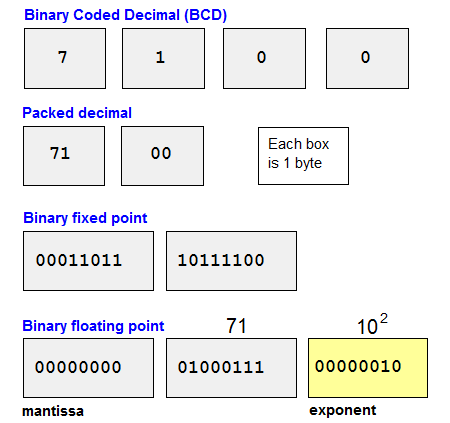# Definition: floating point

A method for storing and calculating numbers in which the decimal points do not line up as in fixed point numbers. The significant digits are stored as a unit called the "mantissa," and the location of the radix point (decimal point in base 10) is stored in a separate unit called the "exponent." Floating point methods are used for calculating a large range of numbers quickly.

Floating point operations can be implemented in software or in a floating point unit (FPU), which may be a separate "math coprocessor" chip or a circuit in the CPU. See math coprocessor, binary numbers and NaN.

```    FLOATING POINT EXAMPLES

Mantissa  Exponent  Value

71        0           71
71        1          710
71        2         7100
71        -1           7.1
```How Numbers Are Stored
Floating point is one of four primary ways numbers are stored in the computer.